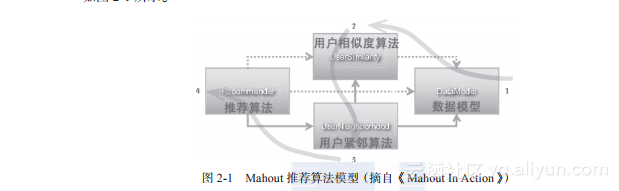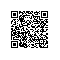# 《R的极客理想——高级开发篇 A》一一2.1　用R重写Mahout协同过滤算法

### 2.1　用R重写Mahout协同过滤算法

2.1.1　Mahout的推荐算法模型org.apache.mahout
mahout-core
0.5

1,101,5.0
1,102,3.0
1,103,2.5
2,101,2.0
2,102,2.5
2,103,5.0
2,104,2.0
3,101,2.5
3,104,4.0
3,105,4.5
3,107,5.0
4,101,5.0
4,103,3.0
4,104,4.5
4,106,4.0
5,101,4.0
5,102,3.0
5,103,2.0
5,104,4.0
5,105,3.5
5,106,4.0

/**

• UserCF测试，单机算法实现程序
*/

public class UserCF {

f?inal static int NEIGHBORHOOD_NUM = 2;        // 取2个近邻
f?inal static int RECOMMENDER_NUM = 3;        // 保留3个推荐结果

public static void main(String[] args) throws IOException, TasteException {
String f?ile = "item.csv";            // 读入数据集
DataModel model = new FileDataModel(new File(f?ile)); // 加载数据到内存对象DataModel
UserSimilarity user = new EuclideanDistanceSimilarity(model);
// 定义用户相似度距离
NearestNUserNeighborhood neighbor = new NearestNUserNeighborhood
(NEIGHBORHOOD_NUM, user, model);    // 定义近邻
Recommender r = new GenericUserBasedRecommender(model, neighbor, user);
// 创建推荐模型
LongPrimitiveIterator iter = model.getUserIDs();    // 取得用户列表

while (iter.hasNext()) {            // 循环用户列表，计算给每个用户的推荐结果
long uid = iter.nextLong();
List list = r.recommend(uid, RECOMMENDER_NUM);
System.out.printf("uid:%s", uid);
for (RecommendedItem ritem : list) {
System.out.printf("(%s,%f)", ritem.getItemID(), ritem.getValue());
// 打印推荐结果
}
System.out.println();
}
}

}

uid:1(104,4.250000)(106,4.000000)
uid:2(105,3.956999)
uid:3(103,3.185407)(102,2.802432)
uid:4(102,3.000000)
uid:5

2.1.2　R语言模型实现

（1）建立数据模型
（2）欧氏距离相似度算法
（3）用户近邻算法
（4）推荐算法
（5）运行程序
1.?建立数据模型

FileDataModel<-function(f?ile){ # 数据模型函数
names(data)<-c("uid","iid","pref") # 增加列名

user <- unique(data$uid) # 计算用户数 item <- unique(sort(data$iid)) # 计算产品数
uidx <- match(data$uid, user) iidx <- match(data$iid, item)
M <- matrix(0, length(user), length(item)) # 定义存储矩阵
i <- cbind(uidx, iidx, pref=data\$pref)

for(n in 1:nrow(i)){ # 给矩阵赋值

M[i[n,],i[n,]]<-i[n,]

}

dimnames(M)[]<-item
M # 返回矩阵数据
}
2.?欧氏距离相似度算法

DistanceSimilarity()，加载用户物品的矩阵数据，通过欧氏距离来计算用户的相似度。
EuclideanDistanceSimilarity<-function(M){
row<-nrow(M)
s<-matrix(0, row, row) # 相似度矩阵

for(z1 in 1:row){

for(z2 in 1:row){
if(z1<z2){
num<-intersect(which(M[z1,]!=0),which(M[z2,]!=0))    # 可计算的列

sum<-0
for(z3 in num){
sum<-sum+(M[z1,][z3]-M[z2,][z3])^2
}

s[z2,z1]<-length(num)/(1+sqrt(sum))

if(s[z2,z1]>1) s[z2,z1]<-1        # 对算法的阈值进行限制
if(s[z2,z1]<-1) s[z2,z1]<- -1
}
}

}

ts<-t(s) # 补全三角矩阵
w<-which(upper.tri(ts))
s[w]<-ts[w]
s # 返回用户相似度矩阵
}
3.?用户近邻算法

NearestNUserNeighborhood<-function(S,n){

row<-nrow(S)
neighbor<-matrix(0, row, n)
for(z1 in 1:row){
for(z2 in 1:n){
m<-which.max(S[,z1])
neighbor[z1,][z2]<-m
S[,z1][m]=0
}
}
neighbor                # 返回前n个最近邻

}
4.?推荐算法

UserBasedRecommender<-function(uid,n,M,S,N){

row<-ncol(N)
col<-ncol(M)
r<-matrix(0, row, col)
N1<-N[uid,]
for(z1 in 1:length(N1)){
num<-intersect(which(M[uid,]==0),which(M[N1[z1],]!=0))   # 可计算的列
for(z2 in num){
r[z1,z2]=M[N1[z1],z2]*S[uid,N1[z1]]
}
}

# print(r)                # 打印每个用户的推荐矩阵

sum<-colSums(r)
s2<-matrix(0, 2, col)
for(z1 in 1:length(N1)){
num<-intersect(which(colSums(r)!=0),which(M[N1[z1],]!=0))
for(z2 in num){
s2[1,][z2]<-s2[1,][z2]+S[uid,N1[z1]]
s2[2,][z2]<-s2[2,][z2]+1
}
}

s2[,which(s2[2,]==1)]=10000
s2<-s2[-2,]

r2<-matrix(0, n, 2)
rr<-sum/s2
item <-dimnames(M)[]
for(z1 in 1:n){
w<-which.max(rr)
if(rr[w]>0.5){
r2[z1,1]<-item[which.max(rr)]
r2[z1,2]<-as.double(rr[w])
rr[w]=0
}
}
r2

}

5.?运行程序

FILE<-"item.csv" # 数据文件
NEIGHBORHOOD_NUM<-2 # 取2个最大近邻
RECOMMENDER_NUM<-3 # 保留最多3个推荐结果

M<-FileDataModel(FILE) # 把数据文件，换成矩阵加载到内存
S<-EuclideanDistanceSimilarity(M) # 计算用户相似度矩阵
N<-NearestNUserNeighborhood(S,NEIGHBORHOOD_NUM) 　# 计算用户近邻

R1<-UserBasedRecommender(1,RECOMMENDER_NUM,M,S,N);R1 　# 查看对User=1的推荐结果

 [,1]  [,2]

[1,] "104" "4.25"

[2,] "106" "4"
[3,] "0" "0"

R2<-UserBasedRecommender(2,RECOMMENDER_NUM,M,S,N);R2 　# 查看对User=2的推荐结果

 [,1]  [,2]

[1,] "105" "3.95699903407931"

[2,] "0" "0"
[3,] "0" "0"

R3<-UserBasedRecommender(3,RECOMMENDER_NUM,M,S,N);R3 　# 查看对User=3的推荐结果

 [,1]  [,2]

[1,] "103" "3.18540697329411"

[2,] "102" "2.80243217111765"
[3,] "0" "0"

R4<-UserBasedRecommender(4,RECOMMENDER_NUM,M,S,N);R4 　# 查看对User=4的推荐结果

 [,1]  [,2]

[1,] "102" "3"

[2,] "0" "0"
[3,] "0" "0"

R5<-UserBasedRecommender(5,RECOMMENDER_NUM,M,S,N);R5 　# 查看对User=5的推荐结果

 [,1] [,2]

[1,] 0 0

[2,] 0 0
[3,] 0 0

2.1.3　算法实现的原理——矩阵变换

1,101,5.0
1,102,3.0
1,103,2.5
2,101,2.0
2,102,2.5
2,103,5.0
2,104,2.0
3,101,2.5
3,104,4.0
3,105,4.5
3,107,5.0
4,101,5.0
4,103,3.0
4,104,4.5
4,106,4.0
5,101,4.0
5,102,3.0
5,103,2.0
5,104,4.0
5,105,3.5
5,106,4.0

 101 102 103 104 105 106 107

[1,] 5.0 3.0 2.5 0.0 0.0 0 0
[2,] 2.0 2.5 5.0 2.0 0.0 0 0
[3,] 2.5 0.0 0.0 4.0 4.5 0 5
[4,] 5.0 0.0 3.0 4.5 0.0 4 0
[5,] 4.0 3.0 2.0 4.0 3.5 4 0

     [,1]      [,2]      [,3]      [,4]      [,5]

[1,] 0.0000000 0.6076560 0.2857143 1.0000000 1.0000000
[2,] 0.6076560 0.0000000 0.6532633 0.5568464 0.7761999
[3,] 0.2857143 0.6532633 0.0000000 0.5634581 1.0000000
[4,] 1.0000000 0.5568464 0.5634581 0.0000000 1.0000000
[5,] 1.0000000 0.7761999 1.0000000 1.0000000 0.0000000

   top1 top2

[1,] 4 5
[2,] 5 3
[3,] 5 2
[4,] 1 5
[5,] 1 3

101  102  103  104  105  106  107

4 0 0 0 4.5 0.0 4 0
5 0 0 0 4.0 3.5 4 0

推荐物品  物品得分

[1,] "104" "4.25"
[2,] "106" "4"

2.1.4　算法总结

similar = 1/(1+sqrt( (a-b)^2 + (a-c)^2 )) # 欧氏距离算法
similar = n/(1+sqrt( (a-b)^2 + (a-c)^2 )) # 改进的欧氏距离算法

a, b是被两个用户都打分的物品，也可能是1个或者多个
n是被两个用户都打分的物品的个数